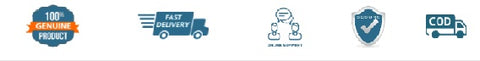# NCERT Mathematics for Class 11 - latest edition as per NCERT/CBSE

SKU:3MNCERT165
Price : Rs. 218.00
Sale price Rs. 218.00 Price : Rs. 242.00
Sold out
Unit price
per
Shipping calculated at checkout.

Chapter 1: Sets
Chapter 2: Relations and Functions
Chapter 3: Trigonometric Functions
Chapter 4: Principle of Mathematical Induction
Chapter 5: Complex Numbers and Quadratic Equations
Chapter 6: Linear Inequalities
Chapter 7: Permutations and Combinations
Chapter 8: Binomial Theorem
Chapter 9: Sequences and Series
Chapter 10: Straight Lines
Chapter 11: Conic Sections
Chapter 12: Introduction to Three Dimensional Geometry
Chapter 13: Limits and Derivatives
Chapter 14: Mathematical Reasoning
Chapter 15: Statistics
Chapter 16: Probability

(All books are 100℅ original and as per the latest NCERT syllabus. Prices includes Rs. 30 per book mandatory binding charges.)## Customer Reviews

Based on 2 reviews
0%
(0)
0%
(0)
50%
(1)
0%
(0)
50%
(1)
K